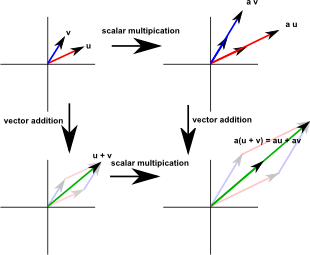# Maths - Euclidean Space

### Euclidean Space Definitions

We can define Euclidean Space in various ways, some examples are:

### Classical Geometry (Euclids Postulates)

This is the traditional approach to geometry known as trigonometry based on points, lines, angles and triangles.

We can distill Euclids postulates down to 5 postulates which define Euclidean Space:

1. A straight line may be drawn from any one point to any other point (any 2 points determine a unique line).
2. A finite straight line may be produced to any length in a straight line.
3. A circle may be described with any centre at any distance from that centre.
4. All right angles are equal.
5. If a straight line meets two other straight lines, so as to make the two interior angles on one side of it together less than two right angles, the other straight lines will meet if produced on that side on which the angles are less than two right angles.

This last postulate, known as the parallel postulate, can be stated in different ways. If we change this, such as allowing more than one line through a point to be parallel to a given line, then we get interesting non-euclidean space defined on this page.

### Euclidean Vector Space

Euclidean space is linear, what does this mean? One way to define this is to define all points on a cartesian coordinate system or in terms of a linear combination of orthogonal (mutually perpendicular) basis vectors.

So any point could be identified by:

P = α Va + β Vb +…

where:

• P = vector representation of a point.
• α, β,… = scalar multipliers (one for each dimension).
• Va, Vb,… = basis vectors (one for each dimension).

So we have two types of mathematical objects: scalars and vectors. The properties of such a space can be defined in terms of vector addition and scalar multiplication as defined by the following axioms:

 Associativity of addition u + (v + w) = (u + v) + w Commutativity of addition v + w = w + v Identity element of addition v + 0 = v Inverse elements of addition −v Distributivity of scalar multiplication with respect to vector addition (u + v)a = ua + av Compatibility of scalar multiplication with field multiplication a(bv) = (ab)v Identity element of scalar multiplication 1v = v

where:

• u,v,w… are vectors
• a,b … are scalarsIn abstract algebra terms we can think of vectors as extensions to the field of real numbers as described on this page.

Euclidean space is quadratic, how can space be both linear and quadratic? We have already seen how vectors and scalar multiplication are linear, some aspects of Euclidean space are quadratic.

For instance the way that we measure distance (the metric) of a space. If we have a two dimensional Euclidean space, where a given point is represented by the vector: v= [x,y] then the distance from the origin is given by the square root of: x² + y². Other physical quantities such as the inertia tensor are also related to the square of the distance to a given point.

### Properties of Euclidean Space

Euclidean space has the following properties:

• There is no preferred origin in euclidean space. Any point would be as good as any other as a choice for the origin.
• There is no preferred direction in euclidean space.
• There is no specific way to define a point at infinity.
• The 'metric' for euclidean space. That is a function, for a given space, that defines the distance between points. For euclidean space, if p and q are two points then:
||p - q||² = (p-q)•(p-q)
• Euclidean space is flat
• Euclidean space is linear
• Euclidean space is continuous (differeniatable)

Euclidean n-space is the most elementary example of an n dimensional manifold.

### Rotations in Euclidean Space

The Cartesian coordinate system allows us to specify directions, but what about direction of rotation? Which direction of rotation do we consider positive? This is an arbitrary decision in that it does not matter as long as we are consistent so, on this website, I have chosen to use the right hand rule. This is because that is the convention used by the VRML/x3d standards.

A rotation can be specified by a vector:If the thumb of the right hand is pointed in the direction of vector, the positive direction of rotation is given by the curl of the fingers.Rotations can be specified in many ways, we could use axis and angle in which case the positive angle direction is as described here. Another alternative is to use Euler Angles where we will use the right hand rule for the positive angle about each base positive coordinate direction.